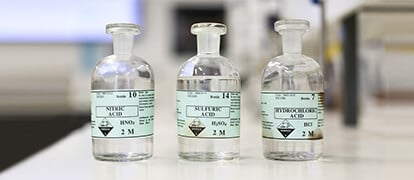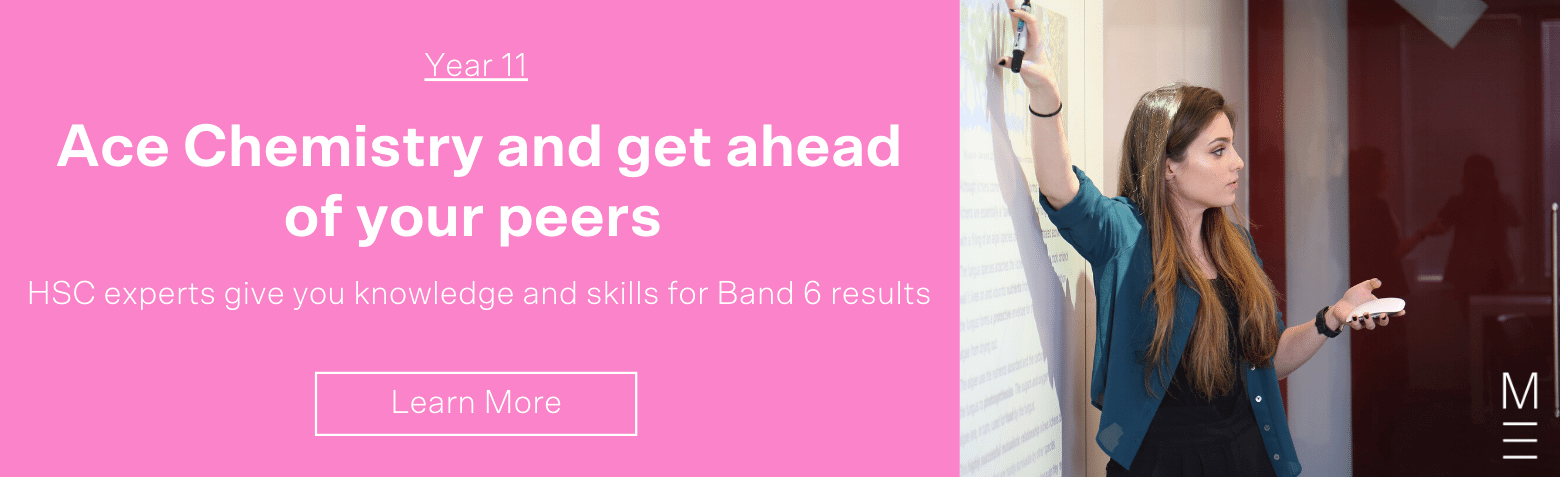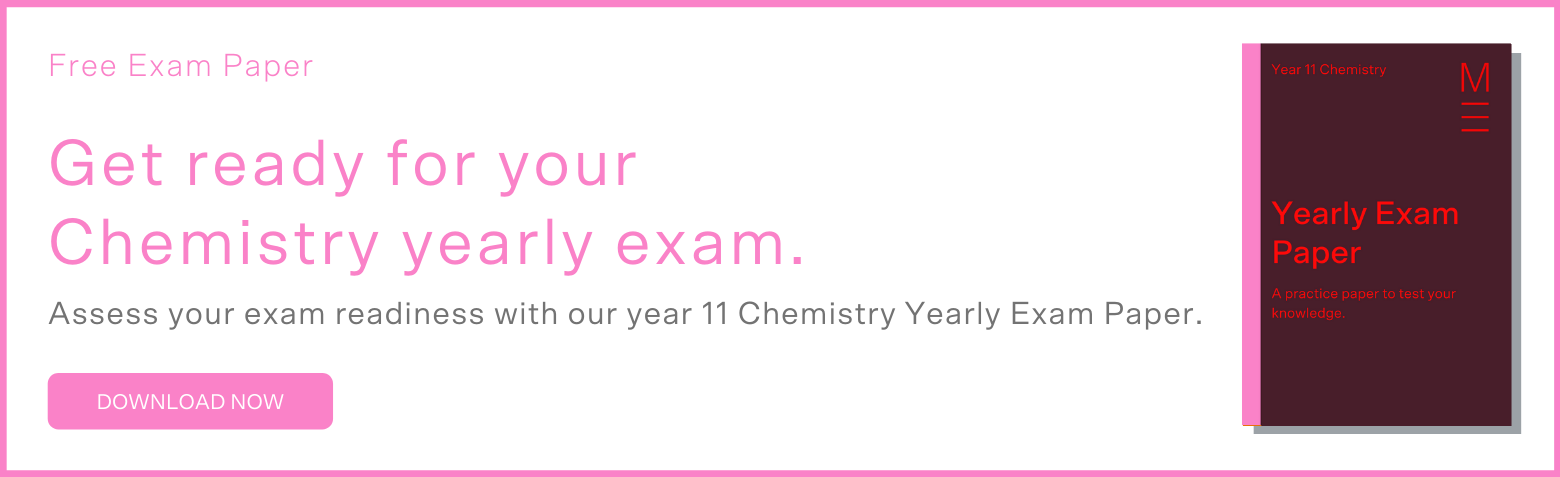# Year 11 Chemistry Practice Questions for Yearly Exam (Free Chemistry Paper Download)## Year 11 Chemistry Practice Paper for Yearly Exam

The Matrix Year 11 Chemistry Yearly Exam Paper contains questions based on the new Year 11 Chemistry syllabus and covers the four modules of the Year 11 Chemistry course:

• Module 1: Properties & Structures of Matter
• Module 2: Introduction to Quantitative Chemistry
• Module 3: Reactive Chemistry
• Module 4: Drivers of Reactions

We have listed below some of the most popular exam questions for your quick reference. You can download the free Matrix Year 11 Chemistry Practice Paper at the bottom of this article.

## Module 1: Properties & Structure of Matter

Commonly asked exam questions on Module 1 Properties & Structures of Matter are:

• Writing the electron configuration of elements and ions in spdf notation
• Explaining the physical properties of substances (metallic, ionic, covalent network, covalent molecular)
• Recalling and explaining periodic trends

### Question 1  (1 mark)

Which VSEPR shape always results in a polar molecule?

(A) Linear

(B) Trigonal Planar

(C) Tetrahedral

(D) Bent

### Question 2  (1 mark)

Write the full electronic configuration of a sodium atom.

### Question 3  (1 mark)

Define the term “electronegativity’.

### Question 4  (3 marks)

Explain why methane has a lower boiling point than water.

## Need more help with Chemistry?

Be one step ahead of your peers with advanced completion of contents before it’s taught at school. You’ll gain an in-depth knowledge and understanding of the key concepts for exam success. Book your free trial for Year 11 Chemistry today and learn more about our Year 11 Chemistry Term Course.## Module 2: Introduction to Quantitative Chemistry

Commonly asked exam questions on Module 1 Properties & Structures of Matter are:

• Completing mole calculations
• Completing limiting reactant calculations
• Recalling and applying gas laws

### Question 5  (1 mark)

Which gas law describes the relationship between pressure and volume?

(A) Boyle’s Law

(B) Charles’ Law

(D) Gay-Lussac’s Law

### Question 6   (5 marks)

3.75 g of potassium carbonate was placed in 280 mL of 0.14 M hydrochloric acid. The following reaction occurs:

K2CO3(aq) + 2HCl(aq) → 2KCl(aq) + CO2(g) + H2O(l)

(A) Write the full ionic equation for this reaction.

(B) Write the net ionic equation for this reaction.

(C) What mass of potassium chloride will be produced?

## Module 3: Reactive Chemistry

Commonly asked exam questions on Module 3 Reactive Chemistry are:

• Classifying and writing equations for different reaction types
• Selecting and combining half-equations for redox reactions
• Calculating cell potentials
• Explaining changes in reaction rates using collision theory

### Question 7  (1 mark)

A galvanic cell was constructed by connecting a graphite electrode in a solution of iron(II) nitrate and iron(III) nitrate, and a tine electrode in a solution of tin(II) chloride.

What is the cell potential under standard conditions?

(A) 0.91 V

(B) 0.63 V

(C) 030 V

(D) 1.68 V

### Question 8  (6 marks)

2.37 g of aluminium was combusted in excess oxygen gas:

4Al(s) + 3O2(g) → 2Al2O3(s)

(a) How many moles of aluminium reacted?

(b) What mass of aluminium oxide was formed?

(c) How many atoms of oxygen reacted?

(d) Explain, with reference to collision theory, how heating up the reaction mixture would increase the rate of this reaction.

(e) Identify two other ways to increase the rate of this reaction.

### Question 9  (2 marks)

Balance the following half-equations by adding coefficients and electrons, and combine them to form a balanced ionic equation.

 C2O42-(aq) → CO2(g) Co3+(aq) → Co2+(aq)

## Module 4: Drivers of Reactions

Commonly asked exam questions on Module 4 Drivers of Reactions are:

• Calculating reaction enthalpies from calorimetric data
• Applying Hess’s Law to energy cycles
• Explaining reaction spontaneity with reference to Gibbs free energy, enthalpy and entropy

### Question 10  (1 mark)

In a calorimetry experiment to determine the enthalpy of solution of lithium hydroxide, the following data were collected:

 Mass of lithium hydroxide 5.73 g Mass of water 265.1 g Initial temperature of water 17.5 °C Final temperature of water 21.6 °C

What is the experimentally determined value of ΔHsoln? The specific heat capacity of the solution is 4.18 x 103 J kg-1 K-1.

(a) − 4.54 kJ mol-1

(b) − 19.4 kJ mol-1

(c) − 4.64 kJ mol-1

(d) − 19.0 kJ mol-1

### Question 11  (1 mark)

Given the following data:

 N2(g) + 3H2(g) → 2NH3(g) ΔH = − 115 kJ mol-1 2NH3(g) + 4H2O(l) → 2NO2(g) + 7H2(g) ΔH = − 142.5 kJ mol-1 H2O(l) → H2(g) + ½O2(g) ΔH = − 43.7 kJ mol-1

What is the enthalpy change for the following reaction?

N2(g) + 2O2(g) → 2NO2(g)

## Are you ready for your upcoming Year 11 Chemistry Yearly Exam?

Test your depth of knowledge and understanding with our 2-hour Year 11 Chemistry Yearly Exam Practice Paper.